Featured

# Line and Spline Chart in ASP.Net

Sometimes we need to show data in a Line Chart, so by considering that requirement and to introduce the ASP.Net Line and Spline Chart controls I have decided to write this article.
Let us learn about the ASP.Net chart types Line and Spline that provide a powerful UI and design quality. So let us start to learn about these chart type controls step-by-step. All the charts are in the System.Web.UI.DataVisualization.Charting namespace.
Chart data is represented using the following points:
1. X Axis: the horizontal line of the chart termed the X axis
2. Y Axis: the vertical line of the chart termed the Y axis
Step 1: Create a table for the chart data
Now before creating the application, let us create a table named orderdet in a database from where we show the records in a chart, the table has the following script (shown in the following image):
```USE [CBS]
GO

/****** Object:  Table [dbo].[QuarterwiseSale]    Script Date: 19-12-2014 00:49:50 ******/
SET ANSI_NULLS ON
GO
SET QUOTED_IDENTIFIER ON
GO

GO

CREATE TABLE [dbo].[QuarterwiseSale](
[id] [int] IDENTITY(1,1) NOT NULL,
[Quarter] [varchar](50) NULL,
[SalesValue] [money] NULL,
CONSTRAINT [PK_QuarterwiseSale] PRIMARY KEY CLUSTERED
(
[id] ASC
)WITH (PAD_INDEX = OFF, STATISTICS_NORECOMPUTE = OFF, IGNORE_DUP_KEY = OFF, ALLOW_ROW_LOCKS = ON, ALLOW_PAGE_LOCKS = ON) ON [PRIMARY]
) ON [PRIMARY]

GO

GO  ```
Now the design will look such as: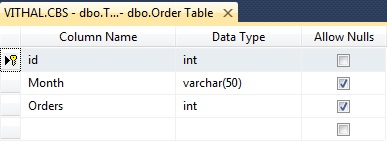Now insert some records as listed in the following image into the table: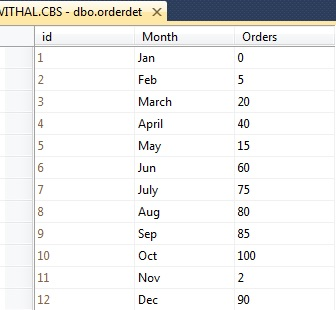I hope you have the same type of table and records as above.
Step 2: Create Web Application

Now create the project
with:
1. "Start" - "All Programs" - "Microsoft Visual Studio 2010".

2. "File" - "New Project" - "C#" - "Empty Project" (to avoid adding a master page).

3. Provide the project a name such as LineAndSpline or another as you wish and specify the location.

4. Then right-click on Solution Explorer and select "Add New Item" then select Default.aspx page.

5. Drag and Drop Chart control from ToolBox onto the Default.aspx page.
Now the Default.aspx source code will be as follows:
```<%@ Page Language="C#" AutoEventWireup="true" CodeFile="Default.aspx.cs" Inherits="_Default" %>

<%@ Register Assembly="System.Web.DataVisualization, Version=4.0.0.0, Culture=neutral, PublicKeyToken=31bf3856ad364e35"
Namespace="System.Web.UI.DataVisualization.Charting" TagPrefix="asp" %>
<!DOCTYPE html PUBLIC "-//W3C//DTD XHTML 1.0 Transitional//EN" "http://www.w3.org/TR/xhtml1/DTD/xhtml1-transitional.dtd">
<html xmlns="http://www.w3.org/1999/xhtml">
<body bgcolor="blue">
<form id="form1" runat="server">
<h4 style="color: White;">
Article for C#Corner
</h4>
<asp:Chart ID="Chart1" runat="server" BackColor="64, 0, 0"
PaletteCustomColors="192, 0, 0" Width="350px">
<Series>
<asp:Series Name="Series1">
</asp:Series>
</Series>
<ChartAreas>
<asp:ChartArea Name="ChartArea1" >
</asp:ChartArea>
</ChartAreas>
<BorderSkin BackColor="" PageColor="192, 64, 0" />
</asp:Chart>

</form>
</body>
</html>  ```
Step 3: Create method to bind the chart control
The Important and complex thing is to bind the chart control properly, because charts exist in a nested series so it's a problem to identify how to set the values.
Now open the default.aspx.cs page and create the following function named Bindchart to bind the Chart Control as in the following:
```private void Bindchart()
{
connection();
query = "select *from Orderdet";//not recommended this i have written just for example,write stored procedure for security
com = new SqlCommand(query, con);
DataSet ds = new DataSet();
da.Fill(ds);

DataTable ChartData=ds.Tables;

//storing total rows count to loop on each Record
string[] XPointMember = new string[ChartData.Rows.Count];
int[] YPointMember = new int[ChartData.Rows.Count];

for (int count = 0; count < ChartData.Rows.Count;count++ )
{
//storing Values for X axis
XPointMember[count] = ChartData.Rows[count]["Month"].ToString();
//storing values for Y Axis
YPointMember[count] = Convert.ToInt32(ChartData.Rows[count]["Orders"]);

}
//binding chart control
Chart1.Series.Points.DataBindXY(XPointMember, YPointMember);

//Setting width of line
Chart1.Series.BorderWidth = 1;
//setting Chart type
Chart1.Series.ChartType = SeriesChartType.Line ;
// Chart1.ChartAreas["ChartArea1"].AxisX.MajorGrid.Enabled = false;
// Chart1.ChartAreas["ChartArea1"].AxisY.MajorGrid.Enabled = false;
//  Chart1.Series.ChartType = SeriesChartType.Spline;
//Chart1.ChartAreas["ChartArea1"].Area3DStyle.Enable3D = true;

con.Close();

}  ```
The entire code of the default.aspx.cs page will look as follows:
```using System;
using System.Collections.Generic;
using System.Linq;
using System.Web;
using System.Web.UI;
using System.Web.UI.WebControls;
using System.Data.SqlClient;
using System.Configuration;
using System.Data;
using System.Web.UI.DataVisualization.Charting;

public partial class _Default : System.Web.UI.Page
{
private SqlConnection con;
private SqlCommand com;
private string constr, query;
private void connection()
{
constr = ConfigurationManager.ConnectionStrings["getconn"].ToString();
con = new SqlConnection(constr);
con.Open();

}
protected void Page_Load(object sender, EventArgs e)
{
if (!IsPostBack)
{
Bindchart();

}
}

private void Bindchart()
{
connection();
query = "select *from Orderdet";//not recommended this i have written just for example,write stored procedure for security
com = new SqlCommand(query, con);
DataSet ds = new DataSet();
da.Fill(ds);

DataTable ChartData=ds.Tables;

//storing total rows count to loop on each Record
string[] XPointMember = new string[ChartData.Rows.Count];
int[] YPointMember = new int[ChartData.Rows.Count];

for (int count = 0; count < ChartData.Rows.Count;count++ )
{
//storing Values for X axis
XPointMember[count] = ChartData.Rows[count]["Month"].ToString();
//storing values for Y Axis
YPointMember[count] = Convert.ToInt32(ChartData.Rows[count]["Orders"]);

}
//binding chart control
Chart1.Series.Points.DataBindXY(XPointMember, YPointMember);

//Setting width of line
Chart1.Series.BorderWidth = 1;
//setting Chart type
Chart1.Series.ChartType = SeriesChartType.Line ;
// Chart1.ChartAreas["ChartArea1"].AxisX.MajorGrid.Enabled = false;
// Chart1.ChartAreas["ChartArea1"].AxisY.MajorGrid.Enabled = false;
//  Chart1.Series.ChartType = SeriesChartType.Spline;
//Chart1.ChartAreas["ChartArea1"].Area3DStyle.Enable3D = true;

con.Close();

}
}  ```
Now, we have the entire logic to bind the chart from the database, let us run the application. The chart will look such as follows: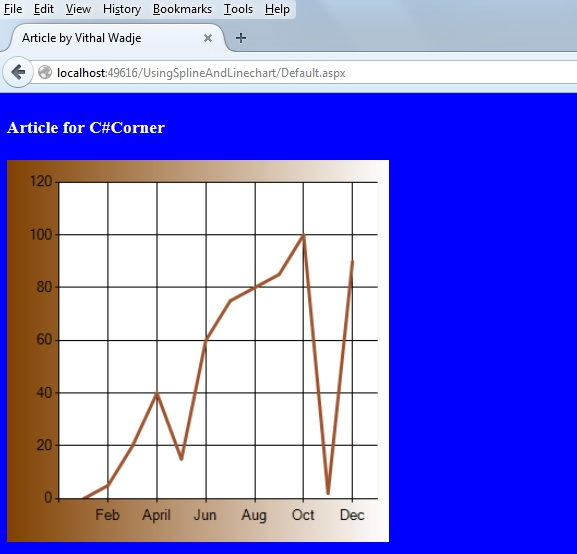We can have GridLines of the chart that sometimes need to be hidden, if you want to hide them then uncomment the following code as:
```Chart1.ChartAreas["ChartArea1"].AxisX.MajorGrid.Enabled = false;
Chart1.ChartAreas["ChartArea1"].AxisY.MajorGrid.Enabled = false; ```
Now run the application, the chart will look as follows: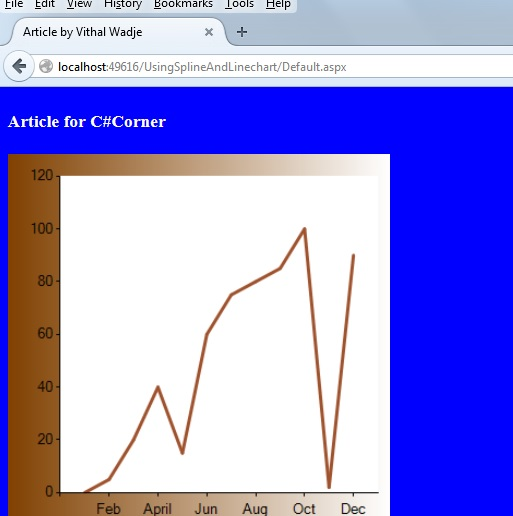We can also change the thickness (width) of the lines as in the following:

```/Setting width of line
Chart1.Series.BorderWidth = 1; ```
Now let's look at the Graph.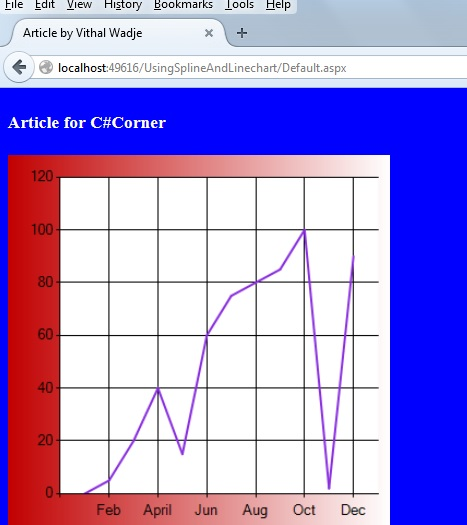Now let us switch to a Spline chart as in the following:
```//setting Chart type
Chart1.Series.ChartType = SeriesChartType.Spline ;  ```
Now run the application and the Funnel type chart will look as follows: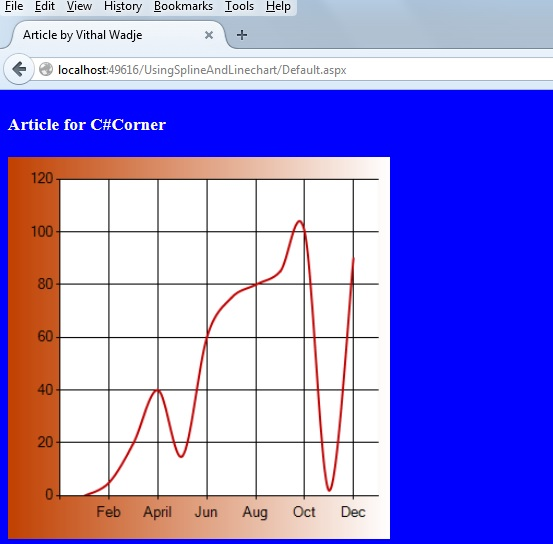The preceding is the Normal Spline chart. Now let us enable the 3D style as in the following:
```/Setting width of line
Chart1.ChartAreas["ChartArea1"].Area3DStyle.Enable3D = true;  ```
Now run the application and the chart will look as follows: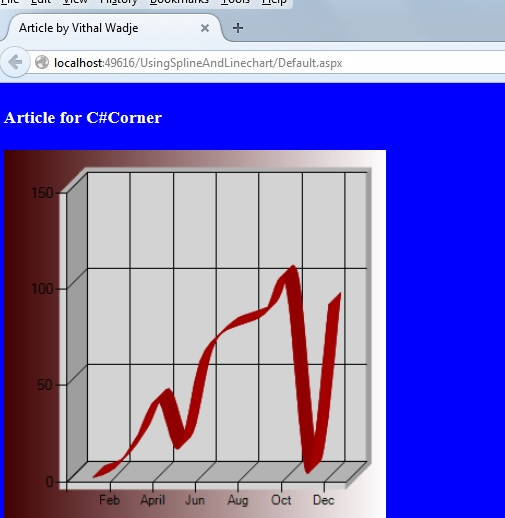Now from all the preceding explanations we saw how to create and use a Line and Spline chart.

Notes
• Change the connection string in the web.config file to specify your server location.
Summary